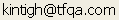Tools for Quantitative Archaeology    Email:EvalC: Evaluate Observed Simpson's C Against Samples Drawn from a Population

EvalC performs a Monte Carlo evaluation of the significance of an observed value of C relative to a given assumption about the population. In it, Monte-Carlo samples with specified sample sizes are drawn with replacement from a population with stipulated type proportions. The program calculates the proportion of Monte Carlo samples drawn from this population that have a C value less than or equal to or greater than or equal to that of the observed sample. Thus, a probability of 0.035 of a random sample having C value as high or higher than the observed C suggests that a sample as concentrated as the observed is unlikely to occur due to sampling error from the stipulated population.

RUNNING EVALC

Input can either come from the keyboard or from a ADF format file. With file input the first observation is taken to represent the population proportions and the subsequent observations, the sample counts. The population can be described by counts or proportions for each of the ntypes types. If counts are entered the program convers them to proportions.

SAMPLE INPUT FILE

```2 5
10 10 20 20 80
3  5  8  6 20```

SAMPLE PROGRAM OUTPUT

```Evaluate Observed Simpson's C Against Samples Drawn from a Population
(c) Keith W. Kintigh, Arizona State University, 2000

Monte-Carlo samples with specified sample sizes are drawn
with replacement from a population with given type proportions.
The program calculates the proportion of Monte Carlo samples
drawn from this population that have a C value less than or
equal to or greater than or equal to that of the observed sample.
Thus, a probability of 0.035 of a random sample having C value
as high or higher than the observed C suggests that a sample as
concentrated as the observed is unlikely to occur due to sampling
error from the stipulated population.

Random Seed: -1799137531
Population Percents (5 Types): Type-Pct
1 -   7.14   2 -   7.14   3 -  14.29   4 -  14.29   5 -  57.14
Population C=0.3776

Type  PopPct  ObsPct  ObsCnt
1    7.14    7.14       3
2    7.14   11.90       5
3   14.29   19.05       8
4   14.29   14.29       6
5   57.14   47.62      20
Observed Sample Size      42   C=0.3027

Pop C=0.3776  Mean Sample C=0.3909  Std. Sample C=0.0686  trials=10000
Obs C=0.3027  p(random<=obs)=0.085500 (855/10000)
p(random>=obs)=0.917200 (9172/10000)
```

 Home Top Overview Ordering Documentation

Page Last Updated - 21-Jul-2007